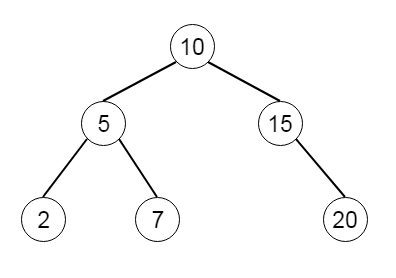# Binary Tree Inorder Traversal in Python

Suppose we have a binary tree. We have to traverse this tree using the inorder traversal scheme without using recursion. So if the tree is likeThen the traversal will be [2,5,7,10,15,20]

To solve this, we will follow these steps −

• Create two array res and stack, set curr := root
• Run one infinite loop
• while current is not null
• push curr into a stack, and set curr := left of curr
• when the length of stack = 0, then return res
• node := popped element from the stack
• insert a value of node into res
• curr := right of curr

## Example

Let us see the following implementation to get a better understanding −

Live Demo

class TreeNode:
def __init__(self, data, left = None, right = None):
self.data = data
self.left = left
self.right = right
def insert(temp,data):
que = []
que.append(temp)
while (len(que)):
temp = que
que.pop(0)
if (not temp.left):
temp.left = TreeNode(data)
break
else:
que.append(temp.left)
if (not temp.right):
temp.right = TreeNode(data)
break
else:
que.append(temp.right)
def make_tree(elements):
Tree = TreeNode(elements)
for element in elements[1:]:
insert(Tree, element)
return Tree
class Solution(object):
def inorderTraversal(self, root):
res, stack = [], []
current = root
while True:
while current:
stack.append(current)
current = current.left
if len(stack) == 0:
return res
node = stack[-1]
stack.pop(len(stack)-1)
if node.data != None:
res.append(node.data)
current = node.right
return res
ob1 = Solution()
root = make_tree([10,5,15,2,7,None,20])
print(ob1.inorderTraversal(root))

## Input

[10,5,15,2,7,null,20]

## Output

[2,5,7,10,15,20]